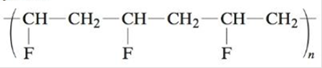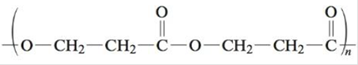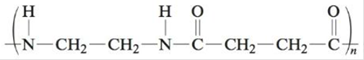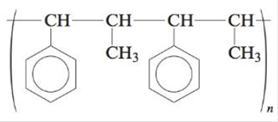Chapter 22, Problem 72E

Chapter
Section
Textbook Problem

# What monomer(s) must be used to produce the following polymers?a.b.c.d.e.f.(This polymer is Kodel, used to make fibers of stain-resistant carpeting.)Classify these polymers as condensation or addition polymers. Which are copolymers?

(a)

Interpretation Introduction

Interpretation: The monomers of the given polymers, their classification (condensation or addition polymers) and copolymers are to be stated.

Concept introduction: The polymers (repeating structural units) are derived from the simple and reactive molecules, called as monomers. Depending upon the mode of polymerization, polymerization mainly occurs by the addition and condensation polymerization reactions. Copolymers are defined as polymers in which repeating structural units are derived from two or more types of monomers.

Explanation

Explanation

To determine: The monomer of the given polymer.

The monomer is vinyl fluoride. It is an addition polymer whose structure is shown in Figure 1.

The structure of the monomer is,

(b)

Interpretation Introduction

Interpretation: The monomers of the given polymers, their classification (condensation or addition polymers) and copolymers are to be stated.

Concept introduction: The polymers (repeating structural units) are derived from the simple and reactive molecules, called as monomers. Depending upon the mode of polymerization, polymerization mainly occurs by the addition and condensation polymerization reactions. Copolymers are defined as polymers in which repeating structural units are derived from two or more types of monomers.

(c)

Interpretation Introduction

Interpretation: The monomers of the given polymers, their classification (condensation or addition polymers) and copolymers are to be stated.

Concept introduction: The polymers (repeating structural units) are derived from the simple and reactive molecules, called as monomers. Depending upon the mode of polymerization, polymerization mainly occurs by the addition and condensation polymerization reactions. Copolymers are defined as polymers in which repeating structural units are derived from two or more types of monomers.

(d)

Interpretation Introduction

Interpretation: The monomers of the given polymers, their classification (condensation or addition polymers) and copolymers are to be stated.

Concept introduction: The polymers (repeating structural units) are derived from the simple and reactive molecules, called as monomers. Depending upon the mode of polymerization, polymerization mainly occurs by the addition and condensation polymerization reactions. Copolymers are defined as polymers in which repeating structural units are derived from two or more types of monomers.

(e)

Interpretation Introduction

Interpretation: The monomers of the given polymers, their classification (condensation or addition polymers) and copolymers are to be stated.

Concept introduction: The polymers (repeating structural units) are derived from the simple and reactive molecules, called as monomers. Depending upon the mode of polymerization, polymerization mainly occurs by the addition and condensation polymerization reactions. Copolymers are defined as polymers in which repeating structural units are derived from two or more types of monomers.

(f)

Interpretation Introduction

Interpretation: The monomers of the given polymers, their classification (condensation or addition polymers) and copolymers are to be stated.

Concept introduction: The polymers (repeating structural units) are derived from the simple and reactive molecules, called as monomers. Depending upon the mode of polymerization, polymerization mainly occurs by the addition and condensation polymerization reactions. Copolymers are defined as polymers in which repeating structural units are derived from two or more types of monomers.

### Still sussing out bartleby?

Check out a sample textbook solution.

See a sample solution

#### The Solution to Your Study Problems

Bartleby provides explanations to thousands of textbook problems written by our experts, many with advanced degrees!

Get Started